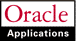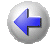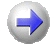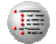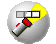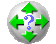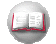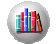Previous Next Contents Index Navigation Glossary Library

## Resizing the Archive Tables

If you are archiving a large number of records for a fiscal year, you can update the FA:Archive Table Sizing Factor to specify the size of the temporary tables created by an archive. Specifically, if the number of rows Oracle Assets will archive multiplied by the average rowsize of that table for all three tables is very different from 100,000 bytes, you may want to adjust the FA: Archive Table Sizing Factor.

Contact your Database Administrator to find out if you need to update this factor. You can tell your Database Administrator that the Sizing Factor specifies how many kilobytes of storage to reserve for the initial extent. The default value is 100.

You can determine approximately how many rows Oracle Assets will archive for a fiscal year using the following SQL script. You can expect to have about six FA_ADJUSTMENTS rows per transaction performed that year, and one FA_DEPRN_DETAIL row and one FA_DEPRN_SUMMARY row for each asset for each period in each book.

To find out about how many FA_ADJUSTMENTS rows Oracle Assets will archive:

```select count(ADJ.ASSET_ID)
FA_DEPRN_PERIODS DP,
FA_FISCAL_YEAR FY
where
FY.FISCAL_YEAR = Fiscal Year To Archive and
DP.CALENDAR_PERIOD_OPEN_DATE >= FY.START_DATE and
DP.CALENDAR_PERIOD_CLOSE_DATE <= FY.END_DATE and

To find out about how many FA_DEPRN_DETAIL rows Oracle Assets will archive:

`select count(DD.ASSET_ID) `
`     from FA_DEPRN_DETAIL DD,`
`          FA_DEPRN_PERIODS DP,`
`          FA_FISCAL_YEAR FY`
`where `
`     FY.FISCAL_YEAR = Fiscal Year To Archive and`
`     DP.CALENDAR_PERIOD_OPEN_DATE >= FY.START_DATE and`
`     DP.CALENDAR_PERIOD_CLOSE_DATE <= FY.END_DATE and`
`     DD.PERIOD_COUNTER = DP.PERIOD_COUNTER;`

To find out about how many FA_DEPRN_SUMMARY rows Oracle Assets will archive:

`select count(DS.ASSET_ID) `
`     from FA_DEPRN_SUMMARY DS,`
`          FA_DEPRN_PERIODS DP,`
`          FA_FISCAL_YEAR FY`
`where `
`     FY.FISCAL_YEAR = Fiscal Year To Archive and`
`     DP.CALENDAR_PERIOD_OPEN_DATE >= FY.START_DATE and`
`     DP.CALENDAR_PERIOD_CLOSE_DATE <= FY.END_DATE and`
`     DS.PERIOD_COUNTER = DP.PERIOD_COUNTER;`

You can determine the average rowsize for each table using something like the following SQL script. You can expect each row to be about 50 bytes.

`select avg(nvl(vsize(column_1),0)) +`
`          avg(nvl(vsize(column_2),0)) +`
`                  . . .`
`          avg(nvl(vsize(column_N),0))`
`     from table_name;`